# NCERT solutions class 9 maths linear equations in two variables 4.3

In this page we have NCERT book Solutions for Class 9th Maths Linear equations in two variables for EXERCISE 4.3 on pages 74 and 75. Hope you like them and do not forget to like , social share and comment at the end of the page.

## Chapter 4 Exercise 4.3

Question 1
Draw the graph of each of the following linear equations in two variables:
(i) x + y = 4
(ii) x - y = 2
(iii) y = 3x
(iv) 3 = 2x + y
Solution
i. To draw the graph, we need at least two solutions of the equation. We can easily find two solutions by putting x=0 and y=0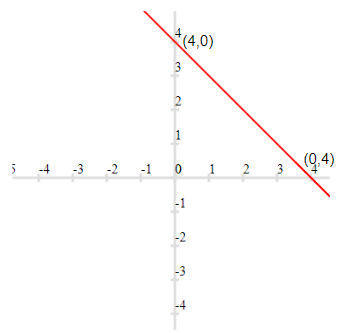ii. To draw the graph, we need at least two solutions of the equation. We can easily find two solutions by putting x=0 and y=0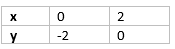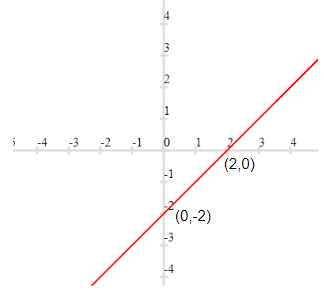iii. To draw the graph, we need at least two solutions of the equation. We can easily find two solutions by putting x=0 and y=0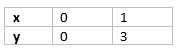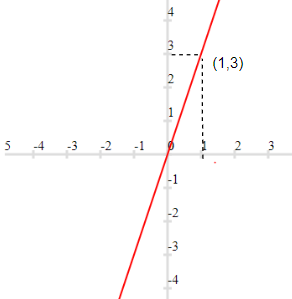iv. To draw the graph, we need at least two solutions of the equation. We can easily find two solutions by putting x=0 and y=0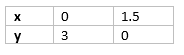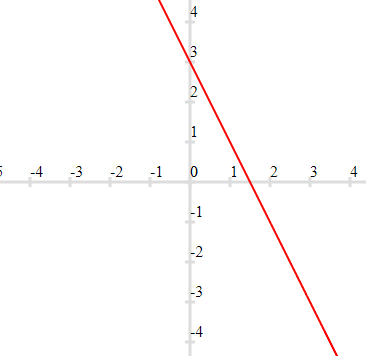Question 2
Give the equations of two lines passing through (2, 14). How many more such lines are there, and why?
Solution
(2, 14) is a point in the coordinate plane.
Two equation of lines which can be pass through are
$7x -y=0$ => $y=7x$ , for x=2 ,y=14
$3x -y + 8$ => $y=8+3x$ , for x= 2, y= 8+6 =14
There can be infinite lines passing through the point

Question 3
If the point (3, 4) lies on the graph of the equation $3y = ax + 7$, find the value of a.
Solution
If the point (3,4) lies on the given line, x=3 and y=4 will satisfy the given equation.
$3y= ax+7$
$3 \times 4= a \times 3+7$
$12 = 3a+7$
$3a=5$
$a= \frac {5}{3}$

Question 4
The taxi fare in a city is as follows: For the first kilometre, the fare is Rs 8 and for the subsequent distance it is Rs 5 per km. Taking the distance covered as x km and total fare as Rs y, write a linear equation for this information, and draw its graph.
Solution
Total fare will be given as = 5(x-1) + 8 = 5x +3
Therefore
$y=5x+3$
Now for Graph, we can takes points x=1 and x=2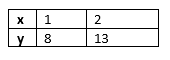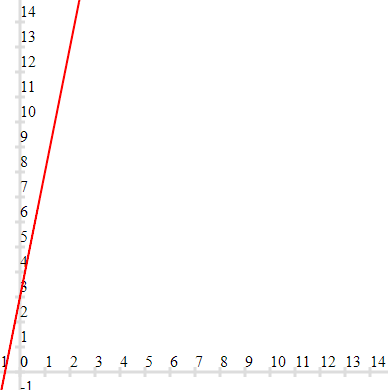Question 5
From the choices given below, choose the equation whose graphs are given in Fig. 4.6 and Fig. 4.7.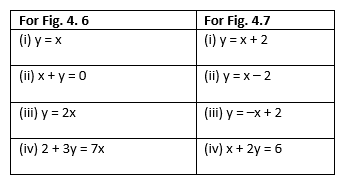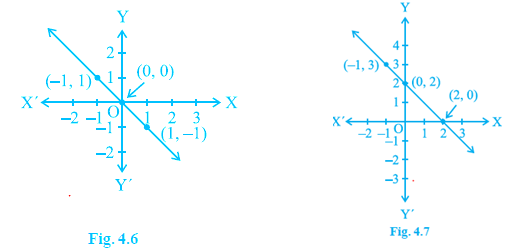Solution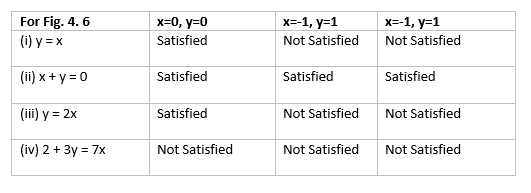So, Answer is $x+y=0$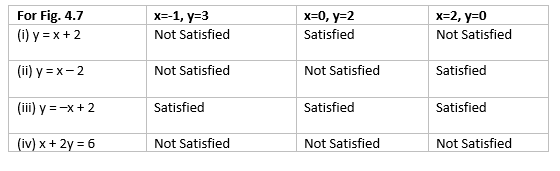So, Answer is $y = -x + 2$

Question 6
If the work done by a body on application of a constant force is directly proportional to the distance travelled by the body, express this in the form of an equation in two variables and draw the graph of the same by taking the constant force as 5 units. Also read from the graph the work done when the distance travelled by the body is (i) 2 units (ii) 0 unit
Solution
Let Work done= y units and distance= x units
Constant force=5 units
It is given
$y= kx$
Now as per question k=5 units, So equation is
$y= 5x$.
Now for Graph, we can takes points x=0 and x=1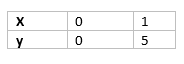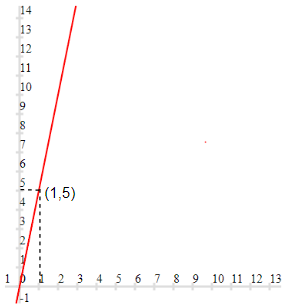Now by reading from graph, we can find the values at x=2 units and x=0 units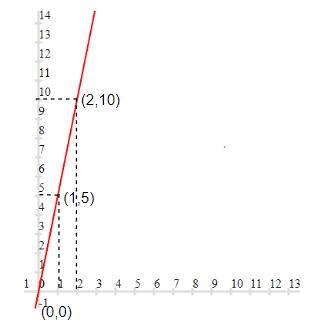x=2 units, y=10 units
x=0 units, y=0 units

Question 7
Yamini and Fatima, two students of Class IX of a school, together contributed Rs 100 towards the Prime Minister�s Relief Fund to help the earthquake victims. Write a linear equation which satisfies this data. (You may take their contributions as Rs x and Rs y.) Draw the graph of the same.
Solution
Linear equation which satisfies would be
$x + y =100$
Now for Graph, we can takes points x=0 and x=100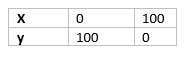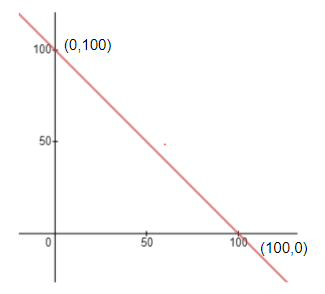Question 8
In countries like USA and Canada, temperature is measured in Fahrenheit, whereas in countries like India, it is measured in Celsius. Here is a linear equation that converts Fahrenheit to Celsius:
$F =\frac {9}{5} C + 32$
(i) Draw the graph of the linear equation above using Celsius for x-axis and Fahrenheit for y-axis.
(ii) If the temperature is 30°C, what is the temperature in Fahrenheit?
(iii) If the temperature is 95°F, what is the temperature in Celsius?
(iv) If the temperature is 0°C, what is the temperature in Fahrenheit and if the temperature is 0°F, what is the temperature in Celsius?
(v) Is there a temperature which is numerically the same in both Fahrenheit and Celsius? If yes, find it.
Solution
Let x be the celsius scale and y be the Fahrenheit scale, then
$y =\frac {9}{5} x + 32$
i. Now for Graph, we can takes points x=0 and x=10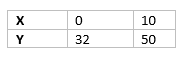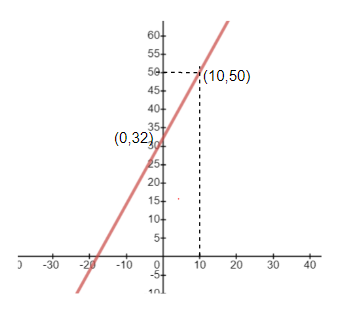ii. $y =\frac {9}{5} x + 32$
For x=30
$y= \frac {9}{5} \times 30 + 32= 86$
So , for 30°C, the temperature in Fahrenheit 86° F

iii. $y =\frac {9}{5} x + 32$
for y = 95
$95 = \frac {9}{5} x + 32$
$\frac {9}{5} x = 95 -32= 63$
$x= \frac {5}{9} \times 63 = 35$
So , for 95° F, the temperature in Celsius 35° C

iv. For x=0
$y= \frac {9}{5} \times 0 + 32= 32$
So , for 0°C, the temperature in Fahrenheit 32° F
For y=0
$0 = \frac {9}{5} x + 32$
$x= -17.8$
So , for 0° F, the temperature in Celsius -17.8 ° C

iv. Let a be the temperature which is numerically the same in both Fahrenheit and Celsius
$a= \frac {9}{5} a + 32$
$5a=9a + 160$v $4a=-160$
$a=-40$
So , for -40° F, the temperature in Celsius -40 ° C

## Summary

1. NCERT solutions for class 9 maths chapter 4 Exercise 4.1 has been prepared by Expert with utmost care. If you find any mistake.Please do provide feedback on mail.
2. This chapter 4 has total 4 Exercise 4.1 ,4.2,4.3 and 4.4. This is the third exercise in the chapter.You can explore previous exercise of this chapter by clicking the link below